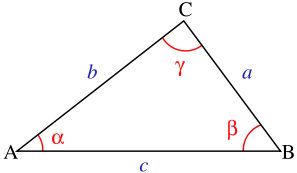From wikipedia:In trigonometry, the law of cosines (also known as the cosine formula or cosine rule) relates the lengths of the sides of a triangle to the cosine of one of its angles. Using notation as in the figure, the law of cosines states

• $c^2~=~a^2~+~b^2~-~2ab\cos\gamma\,$
where $\gamma$ denotes the angle contained between sides of lengths $a$ and $b$ and opposite the side of length $c.$

The law of cosines generalizes Pythagoras' theorem, which holds only for right triangles: if the angle $\gamma$ is a right angle (of measure 90° or $\pi/2$ radians), then $\cos(\gamma)~=~0,$ and thus the law of cosines reduces to Pythagoras' theorem:

• $c^2=a^2+b^2$
The law of cosines is useful for computing the third side of a triangle when two sides and their enclosed angle are known, and in computing the angles of a triangle if all three sides are known.
(none) (none) (none)
(none) (none)

## You are here

CosineRule
PythagorasTheorem